## Table of ContentOpen Access

ARTICLE

# A Theoretical Investigation of the SARS-CoV-2 Model via Fractional Order Epidemiological Model

Tahir Khan1, Rahman Ullah1, Thabet Abdeljawad2,3,*, Manar A. Alqudah4, Faizullah Faiz5

1 Department of Mathematics and Statistics, Woman University, Swabi, Khyber Pakhtunkhwa, 23501, Pakistan
2 Department of Mathematics and Sciences, Prince Sultan University, Riyadh, 11586, Saudi Arabia
3 Department of Medical Research, China Medical University, Taichung, 40402, Taiwan
4 Department of Mathematical Sciences, Faculty of Sciences, Princess Nourah Bint Abdulrahman University, P. O. Box 84428, Riyadh, 11671, Saudi Arabia
5 Department of Basic Sciences and Humanities, College of Electrical and Mechanical Engineering, National University of Sciences and Technology (NUST), Islamabad, 44320, Pakistan

* Corresponding Author: Thabet Abdeljawad. Email:Computer Modeling in Engineering & Sciences 2023, 135(2), 1295-1313. https://doi.org/10.32604/cmes.2022.022177

## Abstract

We propose a theoretical study investigating the spread of the novel coronavirus (COVID-19) reported in Wuhan City of China in 2019. We develop a mathematical model based on the novel corona virus's characteristics and then use fractional calculus to fractionalize it. Various fractional order epidemic models have been formulated and analyzed using a number of iterative and numerical approaches while the complications arise due to singular kernel. We use the well-known Caputo-Fabrizio operator for the purposes of fictionalization because this operator is based on the non-singular kernel. Moreover, to analyze the existence and uniqueness, we will use the well-known fixed point theory. We also prove that the considered model has positive and bounded solutions. We also draw some numerical simulations to verify the theoretical work via graphical representations. We believe that the proposed epidemic model will be helpful for health officials to take some positive steps to control contagious diseases.

## Keywords

1  Introduction

Corona-viruses family causes illnesses in humans, starting with the usual cold and leading to SARS. In the previous twenty years, two corona-virus epidemics have been reported . One of them is SARS, which caused a large epidemic scale in various countries. This epidemic suffered approximately 8000 individuals with 800 deaths. Another type of this virus was the Middle East Respiratory Syndrome Coronavirus (MERS), initially reported in Saudi Arabia and then spreads to many countries, from which 2,500 cases were reported with 800 deaths and still the cause of sporadic cases . A severe outbreak of respiratory illness was reported in Wuhan, China, in December 2019 . The causative agent was identified and isolated from a single patient in early January 2020, the novel coronavirus (COVID-19). The scientific evidence indicated that the first source of transmission of the virus was an animal, while most cases rose due to the contact of infected humans with susceptible humans. The spread of this virus is a burning issue, which reached almost every nook of the World and therefore been reported in more than 200 countries. According to the current statistics, there are more than 494,504,712 confirmed cases, while 6,185,114 deaths occurred till 6th April 2022. It is a public health emergency declared by the World Health Organization (WHO). According to the severeness of this disease, the World Health Organization (WHO) announced it is a public health emergency for international concern. This virus seems to be very contagious, spreading very quickly to almost all over the world and therefore declaring it is a worldwide pandemic. It means that it is a severe public health risk, whose symptoms after infection include cough, fever, fatigue, breathing difficulties, etc.

Fractional computing is a growing field of applied mathematics and has attracted the attention of several researchers . This analysis has widely been utilized to express the axioms of heritage and re-call different physical situations that occur in various fields of applied science. Many classical models are less accurate in predicting, while models with non-integer order are better for allocating and preserving the information that is missing . Moreover, the derivative with classical order does not give the dynamics between two various points [16,17]. It could also be noted that the comparison of classical and fractional order epidemic models reveals that epidemiological models having non-integer order are the generalization of integer order. And so provide more accurate dynamics than classical order, (see for further detail ). A non-integer order model representing the complex dynamics of a biological system has been studied by Ali et al. . Another study used a fractional-order model to explore toxoplasmosis dynamics in human and feline populations . The stability analysis for the spread of pests in tea has been proposed using a fractional-order epidemic model . Similarly, numerous authors studied various dynamical systems with fractional derivatives, e.g., Hadamard and Caputo, Rieman and Liouville . For the solution of Caputo fractional-order epidemiological models, many iterative and numerical methods have been developed due to singular kernel complications arising. So, Caputo et al. presented an idea based on the non-singular kernel to overcome the limitation that arises in the above fractional-order derivatives .

Corona-virus disease (COVID-2019) has been recognized as a global threat and therefore got the attention of various researchers due to its novel nature. Modelling the dynamics of multiple infections disease has a rich literature . A variety of mathematical models have been formulated to study the complicated dynamics of infectious disease and suggest optimal solutions for its future forecast . The novel corona also has rich literature in which the formulation of models and forecasting its future dynamics are investigated . For example, Wu et al. introduced a model to describe the transmission of the disease, based on reported data from 31.12.2019 to 28.01.2020 . Imai et al.  studied the transmission of the disease with the help of computational modeling to estimate the disease outbreak in Wuhan, whose primary focus was on human-to-human transmission. Another study has been investigated by Zhu et al.  to analyze the infectivity of the novel coronavirus. The reported studies indicate that bats and minks may be two animal hosts of the novel coronavirus. Similarly, many more studies have been reported on the dynamics of a novel coronavirus, for instance, see [42,43]. Li et al.  organized a State-of-the-Art Survey using deep learning applications for COVID-19 analysis. Nevertheless, the work proposed by various researchers found in the literature is an excellent contribution; however, it could be improved by incorporating multiple essential and exciting factors related to the newly reported disease of coronavirus transmission.

It could be noted that the coronavirus disease spreading rises globally from human-to-human transmission, while the initial source of the disease was an animal/reservoir. It has also been confirmed from the characteristic of SARS-CoV-2 that various phases of the infections are very significant and influence the transmission of the disease. The latent individuals are notable because of having no symptoms while transmitting the infection. So a small number of latent individuals leads to a significant disaster. We formulate the model keeping in view the above aesthetic of the SARS-CoV-2 virus and study the temporal dynamics of the disease. Once to develop the model, we then fractionalize because of increased development that the epidemiological models having fractional order are more significant than integer-order. Therefore, the fractionalization of the model to its associated fractional-order version will be accorded with the application of fractional calculus. We prove the existence with uniqueness and discuss the feasibility of the developed epidemic problem with the help of the fixed point theory. We will also investigate whether the proposed model is bounded and possesses positive solutions. We perform the numerical visualization of the analytical results to verify the theocratical parts. We also show the difference between integer and non-integer order epidemiological cases.

2  Model Formulation

We formulate the proposed problem by considering the characteristic of the novel coronavirus disease. We classify the total human population Nh(t) in four various compartments of susceptible, latent, infected, and recovered individuals while assuming the reservoir class symbolized with M(t). We further assume the different transmission routes, i.e., from humans and reservoirs. We assume the following assumption before presenting the model:

a. The proposed model represents the dynamical population problem, so all the variables, parameters, and constants are positive.

b. Three different transmission routes transmit the disease, i.e., from a latent population, infected population to susceptible, and from the reservoir.

c. We assume that individuals with a strong immune system will recover in the latent period.

d. It is also assumed that there are two types of recovery from infection, i.e., naturally and due to treatment.

e. The death rate due to disease is assumed to be only in the infected compartment.

Moreover the prorogation of novel corona virus disease transmission is demonstrated by Fig. 1. Hence the combination of all these above assumptions with the disease propagation depicted in the flowchart lead to the system of non-linear differential equation represented by the following system:

{dSh(t)dt=Λ(β1Lh(t)+γβ2Ih(t)+ψβ3M(t)+d)Sh(t),dLh(t)dt=(β1Lh(t)+γβ2Ih(t)+ψβ3M(t))Sh(t)(γ1+γ2+d)Lh(t),dIh(t)dt=γ1Lh(t)(γ3+d+d1)Ih(t),dRh(t)dt=γ2Lh(t)+γ3Ih(t)dRh(t),dM(t)dt=η1Lh(t)+η2Ih(t)αM(t),(1)

and the initial population sizes are assumed to be

Sh(0)>0,Lh(0)0,Ih(0)0,Rh(0)0,M(0)0.(2)Figure 1: The graph describes the flowchart representing the transfer mechanism of the proposed epidemic problem

In the proposed problem, Λ is the ratio of the newborn. We also denote the disease transmission rates by β1, β2 and β2, which respectively represent the transmission from latent, infected, and reservoir, while γ and ψ are the transmission co-efficient. γ1 is the rate at which latent comes to the infected, while γ2 is the natural recovery rate. The recovery due to treatment is symbolized by γ3, and d is assumed to be the natural mortality rate while d1 is the disease-induced death rate. The parameters η1 and η2 are assumed to be the ratio contributing the virus into the seafood market M from latent and infected populations respectively, while the rate α is the removing rate of the virus from it. Let us assume that ρ is a fractional order parameter, i.e., 0<ρ<1. To extend the system (1) to corresponding fractional version, first we describe a few basic concepts related to fractional calculus. These concepts are helpful to retrieve our goals.

Definition 2.1.  Let T>0, and assume that ϕH1(0,T), if n1<ρ<n, and ρ>0 such that nN, then the derivative in the sense of Caputo as well as the Caputo-Fabrizio with ρ order are give by

CFD0,tρ{φ(t)}=K(ρ)(1ρ)0tφ(z)exp((zt)ρ1ρ)dz,(3)

and

CD0,tρ{φ(t)}=1Γ(ρ+n)0t(tz)1+nρφn(z)dz.(4)

In the above Eqs. (3) and (4), CF and C denote the Caputo-Fabrizio and Caputo. Moreover, t is positive, and K(ρ) symbolizes the normilization function, and K(0)=0=K(1).

Definition 2.2.  If 0<ρ<1 and φ(t), varies with time t, then the integral is described by

RLJ0,tρ{φ(t)}=1Γ(ρ)0t(tz)ρ1φ(z)dz,(5)

is known as the Riemann-Liouville integral and

CFJ0,tρ{φ(t)}=2(2ρ)K(ρ){(1ρ)φ(t)+ρ0tφ(z)dz}.(6)

is said to be the Caputo-Fabrizio-Caputo (CF) integral.

Using the theory of fractional calculus to take the associated fractional version of the considered model. Since ρ is the fractional order parameter, and we assume ρ1=γ1ρ+γ2ρ+dρ and ϱ2=γ3ρ+dρ+d1ρ for the shake of simplicity, therefore the application of the above definitions to the proposed systems gives

{CFD0,tρSh(t)=Λρ(β1ρLh(t)+γρβ2ρIh(t)+ψρβ3ρM(t)+dρ)Sh(t),CFD0,tρLh(t)=(β1ρLh(t)+γρβ2ρIh(t)+ψρβ3ρM(t))Sh(t)ρ1Lh(t),CFD0,tρIh(t)=γ1ρLh(t)ρ2Ih(t),CFD0,tρRh(t)=γ2ρLh(t)+γ3ρIh(t)dρRh(t),CFD0,tρM(t)=η1ρLh(t)+η2ρIh(t)αρM(t).(7)

To discuss the feasibility of the above epidemiological system (7) of fractional order, we discuss the existence as well as uniqueness analysis in the subsequent sections.

2.1 Existence and Uniqueness

We exploit fixed point theory to show the model's existence and uniqueness under-considered, as Equation reported (7). We transform the reported system into its associated integral equations as

{Sh(t)=Sh(0)+CFJ0,tρ{Λρ(β1ρLh(t)+γρβ2ρIh(t)+ψρβ3ρM(t)+dρ)Sh(t)},Lh(t)=Lh(0)+CFJ0,tρ{(β1ρLh(t)+γρβ2ρIh(t)+ψρβ3ρM(t))Sh(t)ρ1Lh(t)},Ih(t)=Ih(0)+CFJ0,tρ{γ1ρLh(t)ρ2Ih(t)},Rh(t)=Rh(0)+CFJ0,tρ{γ2ρLh(t)+γ3ρIh(t)dρRh(t)},M(t)=M(0)+CFJ0,tρ{η1ρLh(t)+η2ρIh(t)αρM(t)}.

We apply the definition of CF integral, which ultimately implies that

Sh(t)=Sh(0)+2(1ρ)K(ρ)(2ρ){Λρ(β1ρLh(t)+γρβ2ρIh(t)+ψρβ3ρM(t)+dρ)Sh(t)}+2ρK(ρ)(2ρ)0t{Λρ(β1ρLh(z)+γρβ2ρIh(z)+ψρβ3ρM(z)+dρ)Sh(z)}dz,Lh(t)=Lh(0)+2(1ρ)(2ρ)K(ρ){(β1ρLh(t)+γρβ2ρIh(t)+ψρβ3ρM(t))Sh(t)ρ1Lh(t)}+2ρK(ρ)(2ρ)0t{(β1ρLh(z)+γρβ2ρIh(z)+ψρβ3ρM(z))Sh(z)ρ1Lh(z)}dz,Ih(t)=Ih(0)+2(1ρ)K(ρ)(2ρ){γ1ρLh(t)ρ2Ih(t)}+2ρ(2ρ)K(ρ)0t{γ1ρLh(z)ϱ2Ih(z)}dz,Rh(t)=Rh(0)+2(1ρ)(2ρ){γ2ρLh(t)+γ3ρIh(t)dρRh(t)}1K(ρ)+2ρK(ρ)(2ρ)0t{γ2ρLh(z)+γ3ρIh(z)dρRh(z)}dz,M(t)=M(0)+2(1ρ)(2ρ){η1ρLh(t)+η2ρIh(t)αρM(t)}1K(ρ)+2ρK(ρ)(2ρ)0t{η1ρLh(z)+η2ρIh(z)αρM(z)}dz.

Let us assume that i, i=1,2,,5 describes the kernels such that

{1(Sh(t),t)=Λρ(β1ρLh(t)+γρβ2ρIh(t)+ψρβ3ρM(t)+dρ)Sh(t),2(Lh(t),t)=(β1ρLh(t)+γρβ2ρIh(t)+ψρβ3ρM(t))Sh(t)ϱ1Lh(t),3(Ih(t),t)=γ1ρLh(t)ϱ2Ih(t),4(Rh(t),t)=γ2ρLh(t)+γ3ρIh(t)dρRh(t),5(Mi(t),t)=η1ρLh(t)+η2ρIh(t)αρM(t).(8)

Theorem 2.1. The kernels i, satisfies axioms of Lipschitz conditions.

Proof 1. We assume that Sh and S1h, Lh and Lh1, Ih and Ih1, Rh and Rh1, and M and M1 are the two functions for the above kernels 1, 2, 3, 4 and 5 respectively, then establishing the system is given by

{1(Sh(t),t)1(Sh1(t),t)={Λρ(β1ρLh(t)+γρβ2ρIh(t)+ψρβ3ρM(t)+dρ)Sh(t)},2(Lh(t),t)2(Lh1(t),t)={(β1ρLh(t)+γρβ2ρIh(t)+ψρβ3ρM(t))Sh(t)ϱ1Lh(t)},3(Ih(t),t)3(Ih1(t),t)={γ1ρLh(t)ϱ2Ih(t)},4(Rh(t),t)4(Rh1(t),t)=γ2ρLh(t)+γ3ρIh(t)dρRh(t),5(M(t),t)5(M(t),t)=η1ρLh(t)+η2ρIh(t)αρM(t),

Upon, the application of Cauchy's inequality leads to

{1(Sh(t),t)1(Sh1(t),t){Λρ(β1ρLh(t)+γρβ2ρIh(t)+ψρβ3ρM(t)+dρ)Sh(t)},2(Lh(t),t)2(Lh1(t),t){(β1ρLh(t)+γρβ2ρIh(t)+ψρβ3ρM(t))Sh(t)ϱ1Lh(t)},3(Ih(t),t)3(Ih1(t),t)γ1ρLh(t)ϱ2Ih(t),4(Rh(t),t)4(Rh1(t),t)γ2ρLh(t)+γ3ρIh(t)dρRh(t),5(M(t),t)5(M(t),t)η1ρLh(t)+η2ρIh(t)αρM(t).

We then obtain recursively the following relations:

Sh(t)=2(2ρ){(1ρ)1(Sh(n1)(t),t)+ρ0t1(Sh(n1)(z),z)dz}1K(ρ),Lh(t)=2(2ρ){(1ρ)2(Lh(n1)(t),t)+ρ0t2(Lh(n1)(z),z)dz}1K(ρ),Ih(t)=2(2ρ){(1ρ)3(Ih(n1)(t),t)+ρ0t3(Ih(n1)(z),z)dz}1K(ρ),Rh(t)=2(2ρ)K(ρ){(1ρ)5(Rn1(t),t)+ρ0t5(Rh(n1)(z),z)dz},M(t)=2(2ρ)K(ρ){(1ρ)6(Mn1(t),t)+ρ0t6(Mh(n1)(z),z)dz}.(9)

The difference of two successive terms with the application of norm and majorizing, one may obtain

Un(t)=Shn(t)Sh1,h(n1)(t)2(1ρ)K(ρ)(2ρ)1(Sh(n1)(t),t)1(Sh1,h(n2)(t),t)+2ρK(ρ)(2ρ)||0t[1(Sh(n1)(z),z)1(Sh1,h(n2)(z),z)]dz||,Wn(t)=Lh(t)Lh1,h(n1)(t)2(1ρ)(2ρ)K(ρ)2(Lh(n1)(t),t)2(Lh1,h(n2)(t),t)+2ρ(2ρ)K(ρ)||0t[2(Lh(n1)(z),z)2(Lh1,a(n2)(z),z)]dz||,Xn(t)=Ihn(t)Ih1,h(n1)(t)2(1ρ)K(ρ)(3ρ)3(Ih(n1)(t),t)3(Ih1,h(n2)(t),t)+2ρ(2ρ)||0t[3(Ih(n1)(z),z)3(Ih1,h(n2)(z),z)]dz||1K(ρ),Yn(t)=Rhn(t)Rh1,h(n1)(t)2(1ρ)K(ρ)(2ρ)4(Rh(n1)(t),t)4(Rh1,h(n2)(t),t)+2ρ(2ρ)K(ρ)||0t[4(Rh(n1)(z),z)4(Rh1,h(n2)(z),z)]dz||,Zn(t)=Mn(t)M1,h(n1)(t)2(1ρ)K(ρ)(2ρ)5(M(n1)(t),t)5(M1,h(n2)(t),t)+2ρ(2ρ)K(ρ)||0t[4(M(n1)(z),z)5(M1,h(n2)(z),z)]dz||,(10)

with

{i=0Ui(t)=Shi(t),i=0Wi(t)=Lhi(t),i=0Xi(t)=Ihi(t),i=0Yi(t)=Rhi(t),i=0Zi(t)=Mi(t)(11)

It could be noted that the kernels i satisfy Lipschitz conditions, therefore we may obtain

Un(t)=Shn(t)Sh1,h(n1)(t)2(1ρ)K(ρ)(2ρ)τ1Sh(n1)(t)Sh1,h(n2)(t)+2ρ(2ρ)K(ρ)τ20t||Sh(n1)(z)Sh1,h(n2)(z)||dz,Wn(t)=Lhn(t)Lh1,h(n1)(t)2(1ρ)(2ρ)K(ρ)τ5Lh(n1)(t)Lh1,h(n2)(t)+2ρK(ρ)(2ρ)τ60t||Lh(n1)(z)Lh1,h(n2)(z)||dz,Xn(t)=Ihn(t)Ih1,h(n1)(t)2(1ρ)(2ρ)K(ρ)τ5Ih(n1)(t)Ih1,h(n2)(t)+2ρK(ρ)(2ρ)τ60t||Ih(n1)(z)Ih1,h(n2)(z)||dz,Yn(t)=Rhn(t)Rh1,h(n1)(t)2(1ρ)K(ρ)(2ρ)τ9Rh(n1)(t)Rh1,h(n2)(t)+2ρ(2ρ)K(ρ)τ100t||Rh(n1)(z)Rh1,h(n2)(z)||dz,Zn(t)=Mn(t)M1,(n1)(t)2(1ρ)K(ρ)(2ρ)τ9Mn1(t)M1,(n2)(t)+2ρ(2ρ)K(ρ)τ100t||Mn1(z)M1,(n2)(z)||dz.(12)

Theorem 2.2. The epidemiological model of fractional order (7) possesses a solution.

Proof 2. From the assertions derived in Eq. (11) with the utilization of recursive formulas we obtain

Un(t)Sh(0)+{2(2ρ)K(ρ)}n{(tρτ2)n+((1ρ)τ1)n},Wn(t)Lh(0)+{2(2ρ)K(ρ)}n{(τ3(1ρ))n}+{(tρτ4)n},Xn(t)Ih(0)+{2(2ρ)K(ρ)}n{(tρτ6)n}+{((1ρ)τ5)n},Yn(t)Rh(0)+{2(2ρ)K(ρ)}n{((1ρ)τ7)n}+{(ρτ8t)n},Zn(t)M(0)+{2(2ρ)K(ρ)}n{((1ρ)τ9)n}+{(ρτ10t)n}.(13)

So, the relations as described by the above equation are smooth and exists, however to investigate that the functions in these relations are the solutions for system (7), we making the substitutions

{Sh(t)=Shn(t)Υ1,n(t),Lh(t)=Lhn(t)Υ2,n(t),Ih(t)=Ihn(t)Υ3,n(t),Rh(t)=Rhn(t)Υ5,n(t),M(t)=Mn(t)Υ6,n(t),(14)

where Υi,n(t), i=1,2,,5 denote remainder terms of the series, thus

Sh(t)Sh(n1)(t)=2(1ρ)1(Sh(t)Υ1,n(t))(2ρ)K(ρ)+2ρK(ρ)(2ρ)0t1(Sh(z)Υ1,n(z))dz,Lh(t)Lh(n1)(t)=22(Lh(t)Υ2,n(t))(1ρ)(2ρ)K(ρ)+2ρK(ρ)(2ρ)0t2(Lh(z)Υ2,n(z))dz,Ih(t)Ih(n1)(t)=2(1ρ)3(Ih(t)Υ3,n(t))(2ρ)K(ρ)+2ρK(ρ)(2ρ)0t3(Ih(z)Υ3,n(z))dz,Rh(t)Rh(n1)(t)=(1ρ)24(Rh(t)Υ4,n(t))K(ρ)(2α)+2α(2ρ)K(ρ)0t4(Rh(z)Υ4,n(z))dz,M(t)M(n1)(t)=(1ρ)25(M(t)Υ5,n(t))K(ρ)(2ρ)+2ρ(2ρ)K(ρ)0t5(M(z)Υ5,n(z))dz.(15)

The application of norm on both sides of the above system with utilization of the Lipschitz axiom gives that

||Sh(t)2ρK(ρ)(2ρ)0t1(Sh(z),z)dz2(1ρ)1(Sh(t),t)(2ρ)K(ρ)Sh(0)||Υ1,n(t){2ρτ2t(2ρ)K(ρ)+(τ12(1ρ)K(ρ)(2ρ))+1},||Lh(t)2ρ(2ρ)K(ρ)0t2(Lh(z),z)dz22(Lh(t),t)(1ρ)K(ρ)(2ρ)Lh(0)||Υ2,n(t){(2τ3(1ρ)(2ρ)K(ρ)+2tτ4ρk(ρ)(2ρ))+1},||Ih(t)23(Ih(t),t)(1ρ)(2ρ)K(ρ)Ih(0)2ρ(2ρ)K(ρ)0t3(Ih(z),z)dz||Υ3,n(t){(2tρτ6(2ρ)K(ρ)+(1ρ)2τ5(2ρ)K(ρ))+1},||Rh(t)24(Rh(t),t)(1ρ)(2ρ)K(ρ)Rh(0)2ρK(ρ)(2ρ)0t4(Rh(z),z)dz||Υ4,n(t){(2τ7(1ρ)(2ρ)K(ρ)+2tτ8ρ(2ρ)K(ρ))+1},||M(t)5(M(t),t)2(1ρ)(2ρ)K(ρ)M(0)2ρK(ρ)(2ρ)0t5(M(z),z)dz||Υ5,n(t){(2ρtτ12K(ρ)(2ρ)+(1ρ)2τ11(2ρ)K(ρ))+1}.(16)

The application of lim without bound, i.e., whenever t tend to leads to

Sh(t)=2K(ρ)(2ρ){(1ρ)1(Sh(t),t)+ρ0t1(Sh(z),z)dz+Sh(0)},Lh(t)=2(2ρ)K(ρ){(1ρ)2(Lh(t),t)+ρ0t2(Ah(z),z)dz+Lh(0)},Ih(t)=2K(ρ)(2ρ){(1ρ)3(Ih(t),t)+ρ0t3(Ih(z),z)dz+Ih(0)},Rh(t)=2K(ρ)(2ρ){(1ρ)4(Rh(t),t)+ρ0t4(Rh(z),z)dz+Rh(0)},M(t)=2K(ρ)(2ρ){(1ρ)5(M(t),t)+ρ0t5(M(z),z)dz+M(0)},(17)

which completes the proof that solutions of the reported model described by Eq. (7) exists.

Theorem 2.3. The model reported by Eq. (7) posses a unique solution.

Proof 3. Let us assume that (Sh+(t),Lh+(t),Ih+(t),Rh+(t),M+(t)) is also a solution of the system (7), then

Sh(t)Sh+(t)=2(1ρ){1(Sh(t),t)1(Sh+(t),t)}K(ρ)(2ρ)+2ρK(ρ)(2ρ)0t{1(Sh(z),z)1(Sh+(z),z)}dz,Lh(t)Lh+(t)={2(Lh(t),t)2(Lh+(t),t)}2(1ρ)(2ρ)K(ρ)+2ρK(ρ)(2ρ)0t{2(Lh(z),z)2(Lh+(z),z)}dz,Ih(t)Ih+(t)=2(1ρ){3(Ih(t),t)3(Ih+(t),t)}K(ρ)(2ρ)+2ρ(2ρ)K(ρ)0t{3(Ih(z),z)3(Ih+(z),z)}dz,Rh(t)Rh+(t)=2(1ρ){4(Rh(t),t)4(Rh+(t),t)}K(ρ)(2ρ)+2ρ(2ρ)K(ρ)0t{4(Rh(z),z)4(Rh+(z),z)}dz,M(t)M+(t)=2(1ρ){5(M(t),t)5(M+(t),t)}K(ρ)(2ρ)+2ρ(2ρ)K(ρ)0t{5(M(z),z)5(M+(z),z)}dz.

Majorizing one may leads to the assertions as given by

Sh(t)Sh+(t)=2(1ρ)1(Sh(t),t)1(Sh+(t),t)K(ρ)(2ρ)+2ρK(ρ)(2ρ)0t1(Sh(z),z)1(Sh+(z),z)dz,Lh(t)Lh+(t)=2(1ρ)2(Lh(t),t)2(Lh+(t),t)K(ρ)(2ρ)+2ρ(2ρ)K(ρ)0t2(Lh(z),z)2(Lh+(z),z)dz,Ih(t)Ih+(t)=2(1ρ)3(Ih(t),t)3(Ih+(t),t)K(ρ)(2ρ)+2ρ(2ρ)K(ρ)0t3(Ih(z),z)3(Ih+(z),z)dz,Rh(t)Rh+(t)=2(1ρ)4(Rh(t),t)4(Rh+(t),t)K(ρ)(2ρ)+2ρ(2ρ)K(ρ)0t4(Rh(z),z)4(Rh+(z),z)dz,M(t)M+(t)=2(1ρ)5(M(t),t)5(M+(t),t)K(ρ)(2ρ)+2ρ(2ρ)K(ρ)0t5(M(z),z)5(M+(z),z)dz.(18)

We now use the result stated by Theorems 2.1 and 2.2, we obtain

Sh(t)Sh+(t)(ρτ22tϕ2(2ρ)K(ρ))n+2τ1ψ1(1ρ)K(ρ)(2ρ),Lh(t)Lh+(t)2τ3(1ρ)ψ3K(ρ)(2ρ)+(ρϕ42τ4t(2ρ)K(ρ))n,Ih(t)Ih+(t)2(1ρ)τ5ψ5(2ρ)K(ρ)+(2ρτ6ϕ6t(2ρ)K(ρ))n,Rh(t)Rh+(t)2τ7ψ7(1ρ)K(ρ)(2ρ)+(2ρτ8ϕ8t(2ρ)K(ρ))n,M(t)M+(t)2τ9ψ9(1ρ)K(ρ)(2ρ)+(2ρτ10ϕ10tK(ρ)(2ρ))n.(19)

For all n, the inequalities as reported by the above Eq. (19) holds, so

Sh(t)=Sh+(t),Lh(t)=Lh+(t),Ih(t)=Ih+(t),Rh(t)=Rh+(t),M(t)=M+(t).(20)

Now we are going to discuss the biological as well as mathematical feasibility of the problem under consideration. Notably, we discuss the positivity and boundedness of the reported model (7) to prove that the under-considered problem is well-possed. We also investigate that the dynamics of the proposed model are confined to a specific region that is invariant positively. The following Lemmas is established for this purpose.

Lemma 2.1. Since (Sh(t),Lh(t),Ih(t),Rh(t),M(t)) is the solution of the proposed model (7) and let us consider that it possessing non-negative initial sizes of population, then (Sh(t),Lh(t),Ih(t),Rh(t),M(t)) are non-negative for all t0.

Proof 4. We assume that, ω is the fractional order for the system (7), then

{GD0,tω(Sh(t))=Λω(β1ωLh(t)+γωβ2ωIh(t)+ψωβ3ωM(t)+dω)Sh(t),GD0,tω(Lh(t))=(β1ωLh(t)+γωβ2ωIh(t)+ψωβ3ωM(t))Sh(t)ϱ1Lh(t),GD0,tω(Ih(t))=γ1ωLh(t)ϱ2Ih(t),GD0,tω(Rh(t))=γ2ωLh(t)+γ3ωIh(t)dωRh(t),GD0,tω(M(t))=η1ωLh(t)+η2ωIh(t)αωM(t),(21)

where G represents the fractional operator having order is ω, so we have

{GD0,tωSh(t)|κ(Sh)=Λω>0,GD0,tωLh(t)|κ(Lh)=(β1ωLh(t)+γωβ2ωIh(t)+ψωβ3ωM(t))Sh(t)0,GD0,tωIh(t)|κ(Ih)=γ1ωLh(t)0,GD0,tωRh(t)|κ(Rh)=γ2ωLh(t)+γ3ωIh(t)0,GD0,tωM(t)|κ(M)=η1ωLh(t)+η2ωIh(t)0,(22)

where κ(ξ)={ξ=0andSh,Lh,Ih,Rh,M is in C(R+×R+)} and ξ{Sh,Lh,Ih,Rh,M}. Following the methodology proposed in , we reach to the conclusion that the solutions are non-negative for all non-negative t.

Lemma 2.2. Let us assume that the Ω is the feasible region of the model (7), then within it the model that is under consideration is invariant positively and

Ω={(Sh(t),Lh(t),Ih(t),Rh(t),M(t))R+5:Sh+Lh+Ih+Rh(Λd)ρandM(t)Λρ(η1ρ+η2ρ)dραρ}.(23)

Proof 5. Let Nh(t) represents the total human population, then the use of proposed fractional model leads to the assertion is give by

GD0,tρNh(t)+dρNh(t)ΛρNh(t).(24)

Solving the above Eq. (24) which looks like

Nh(t)Nh(0)Eω(dρtρ)+(Λd)ρ(1Eρ(dρtρ)).(25)

It could be also noted that Lh,IhNh, so the last equation of the fractional model (7) looks like

GD0,tρM(t)+αρM(t)(η1ρ+η2ρ)Λραρdρ.(26)

The solution of Eq. (26) leads to

M(t)M(0)Eρ(αρtρ)+(Λρ(η1ρ+η2ρ)dραρ)(1Eρ(αρtρ)).(27)

In Eqs. (25) and (27), E(.) denote the Mittag-Leffler function and Eρ(Z)=n=0ZnΓ(ρi+1). Further, it is obvious that when times grows without bound then Eqs. (25) and (27) gives that Nh(t)(Λd)ρ and M(t)(Λ(η1ρ+η2ρ)dραρ). Thus, if Nh(0)(Λd)ρ and M(0)(Λρ(η1ρ+η2ρ)dραρ), then Nh(t)(Λd)ρ and M(t)(Λρ(η1ρ+η2ρ)dραρ) for every t>0, while if Nh(0)>(Λd)ρ and M(0)>(Λρ(η1ρ+η2ρ)dραρ), then Nh and M contained in ρ and will never leave. So, the dynamics of the fractional epidemic model can be investigated in feasible region Ω.

3  Numerical Analysis

We discuss the temporal dynamics of the considered model for the long run and present the significance of the fractional parameter. We find the numerical simulation to verify the theocratical work carried out for the fractional-order SARS-CoV-2 transmission epidemiological model (7). To show the validity of the analytical findings we present the large-scale simulation. There are not many choices like the traditional numerical methods to choose various schemes for the numerical simulation of fractional order models , therefore extensive attention is required to formulate new and convenient techniques for the simulation of fractional models. We follow a numerical scheme formulated in . We assume the time step h=103 for integration with the simulation interval [0, t], n=Th and nN. We also assume that u=0,1,2,,n, therefore the discretization for the proposed model looks like

{CFSh(u+1)=Sh(0)+{Λρ(β1ρLh(t)+γρβ2ρIh(t)+ψρβ3ρM(t)+dρ)Sh(t)}(1ρ)+ρhk=0u{Λρ(β1ρLh(t)+γρβ2ρIh(t)+ψρβ3ρM(t)+dρ)Sh(t)},CFLh(u+1)=Lh(0)+{(β1ρLh(t)+γρβ2ρIh(t)+ψρβ3ρM(t))Sh(t)ϱ1Lh(t)}(1ρ)+ρhk=0u{(β1ρLh(t)+γρβ2ρIh(t)+ψρβ3ρM(t))Sh(t)ϱ1Lh(t)},CFIh(u+1)=(1α){γ1ρLh(t)ϱ2Ih(t)}+αhk=0u{γ1ρLh(t)ϱ2Ih(t)}+Ih(0),CFRh(u+1)=(1ρ){γ2ρLh(t)+γ3ρIh(t)dρRh(t)}+ρhk=0u{γ2ρLh(t)+γ3ρIh(t)dρRh(t)}+Rh(0),CFM(u+1)=(1ρ){η1ρLh(t)+η2ρIh(t)αρM(t)}+M(0)+ρhk=0u{η1ρLh(t)+η2ρIh(t)αρM(t)}.

Furthermore, we have chosen the value of epidemic parameters biologically as given in Table 1. We also assume the initial population sizes for various compartments of the proposed model as (100,90,80,70,60). We use the Matlab software package to execute the model for numerical simulations. The collection of all these data along with the execution of developed scheme for the epidemic problem leads to the results as depicted in Figs. 26, which respectively visualize the temporal dynamics of Sh, Ah, Ih, Qh, Rh and M. Notably, the temporal dynamics of the susceptible are shown in Fig. 2. We note a significant impact of the fractional-order on the transmission dynamics of sensitive individuals that if the fractional parameter ρ, increases, the number of susceptible individuals are decreases as shown in Fig. 2. This guarantees that there is a strong relation between fractional parameter and the dynamics of susceptible individuals. Further, the dynamics of the considered problem describe that the long run for latent, infected and recovered compartmental populations are also influencing with the variation in fractional parameter as shown in Figs. 35, respectively. This reveals that there is a strong influence of the fractional parameter (ρ) on the disease propagation as depicted respectively in Figs. 35. In a similar fashion, the temporal dynamics of the reservoir are depicted in Fig. 6. It could be seen that the parameter, ρ is a direct relation, and so the ratio of the reservoir is increased whenever ρ is increased as presented in Fig. 6. Thus, the above analysis and the comparison with the integer-order ρ=1 investigate that the CF model gives more accurate dynamics of the disease and provide valuable outputs instead of classical models. Also, it could be concluded that integer order models are not appropriate while models with fractional order are more feasible to represent the accurate dynamics of such type of infectious diseases propagation.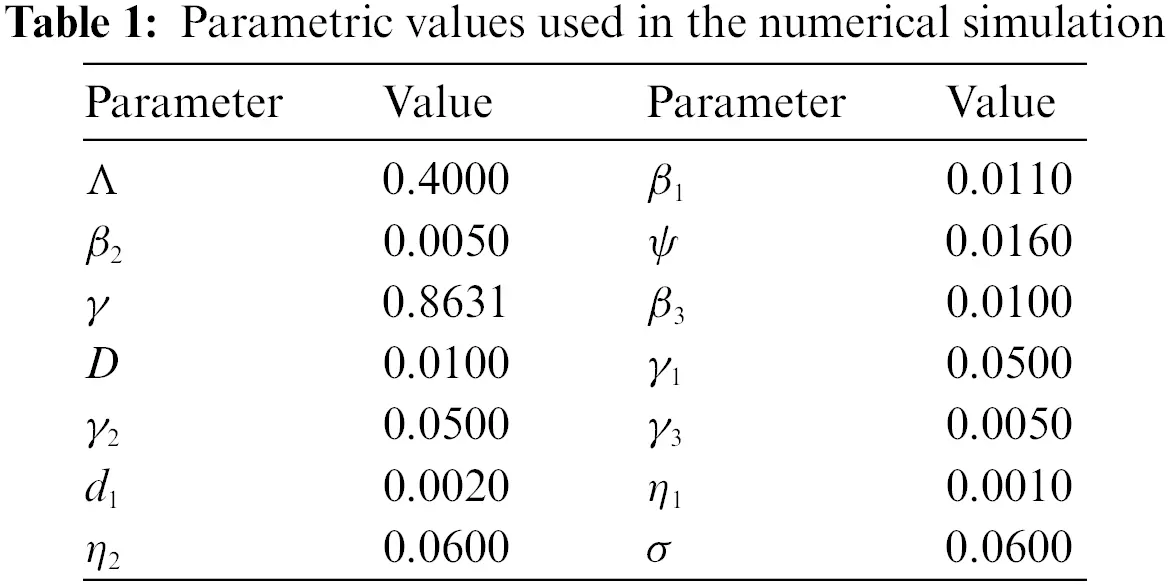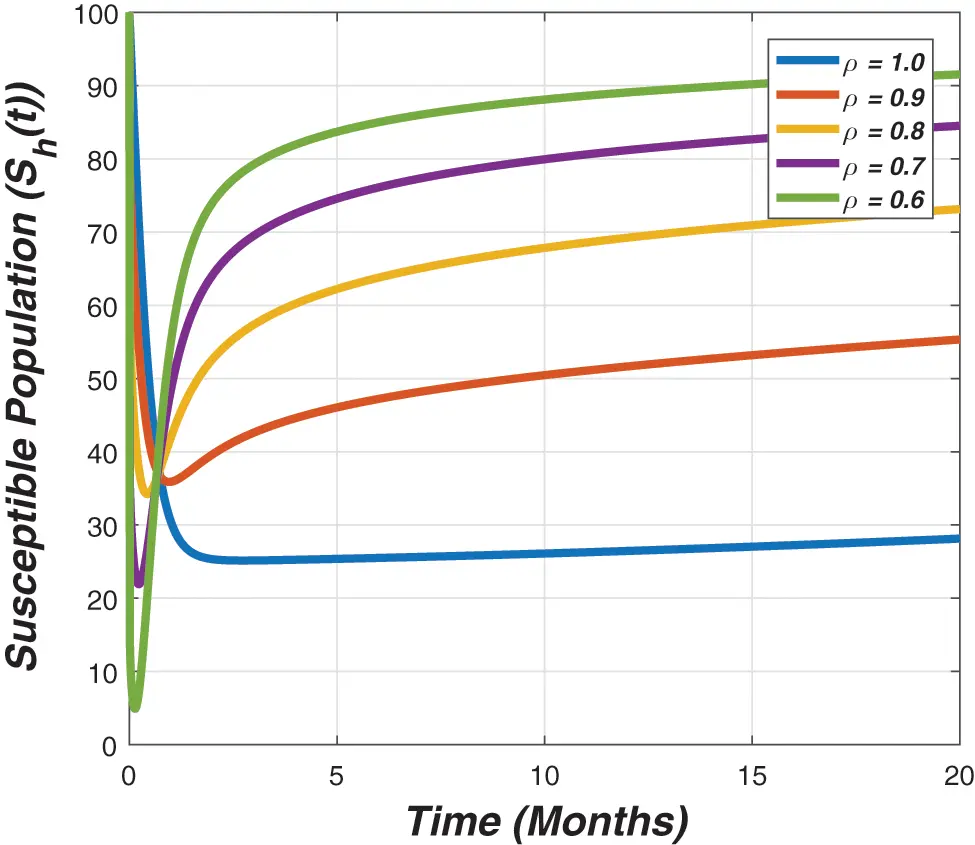Figure 2: The graph visualizes the temporal dynamics of the susceptible for long run against various value of the fractional parameter (ρ) and epidemic parameters given in Table 1. We also used the initial population sizes are as (100,90,80,70,60)Figure 3: The plot demonstrate the dynamical behaviour of the latent individuals against the epidemic parameters value presented in Table 1 and different value of fractional parameter (ρ), while the initial sizes for compartmental population are as (100,90,80,70,60)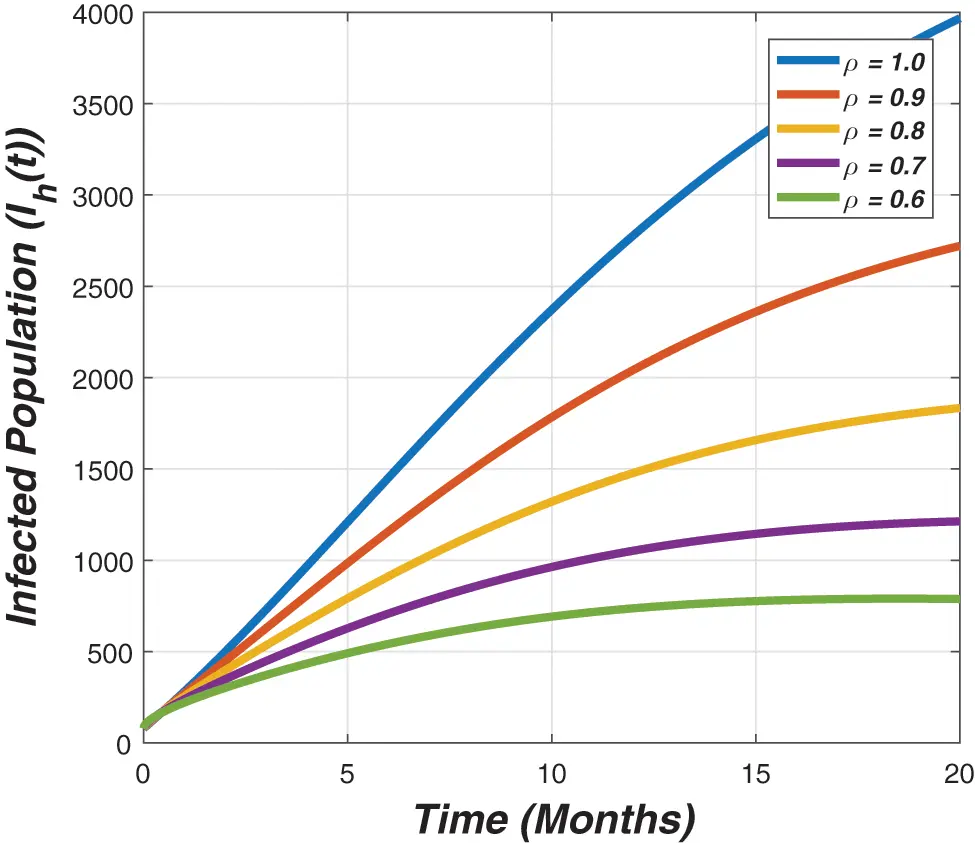Figure 4: The graph represents the temporal dynamics of infected individuals against the fractional parameter (ρ) and model parameters given in Table 1 with initial conditions (100,90,80,70,60)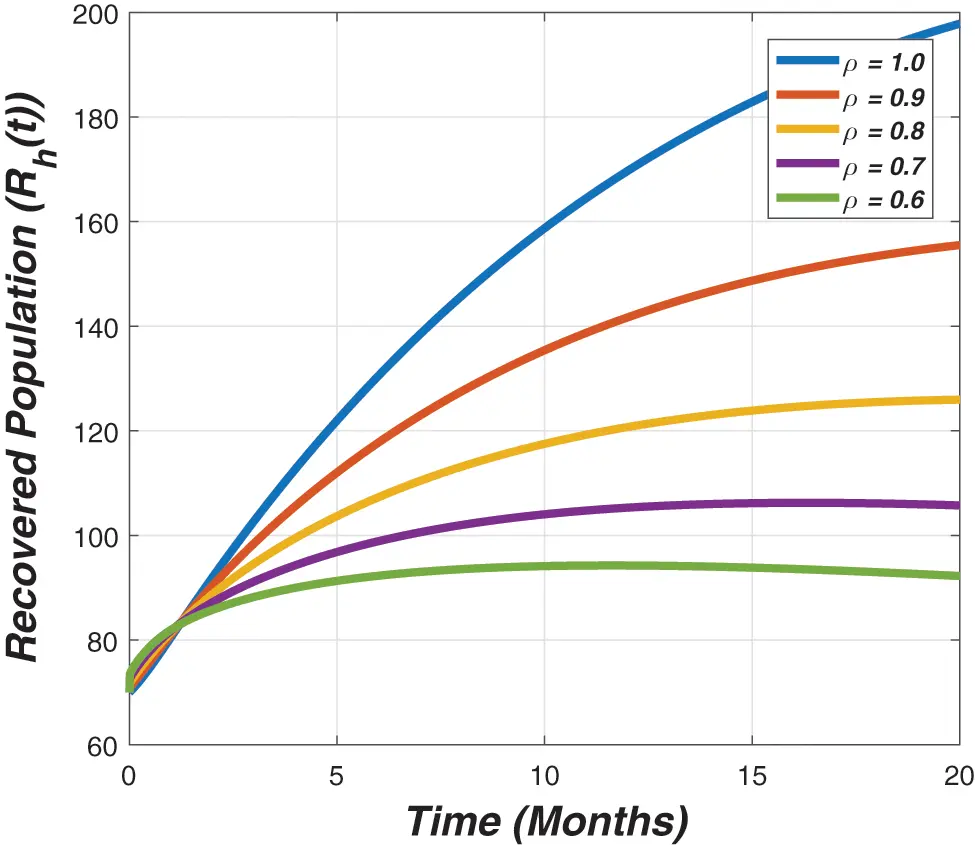Figure 5: The graph describes the dynamics of the recovered individuals for different value of the fractional parameter (ρ) and model parameters given in Table 1, while the initial guess are as (100,90,80,70,60)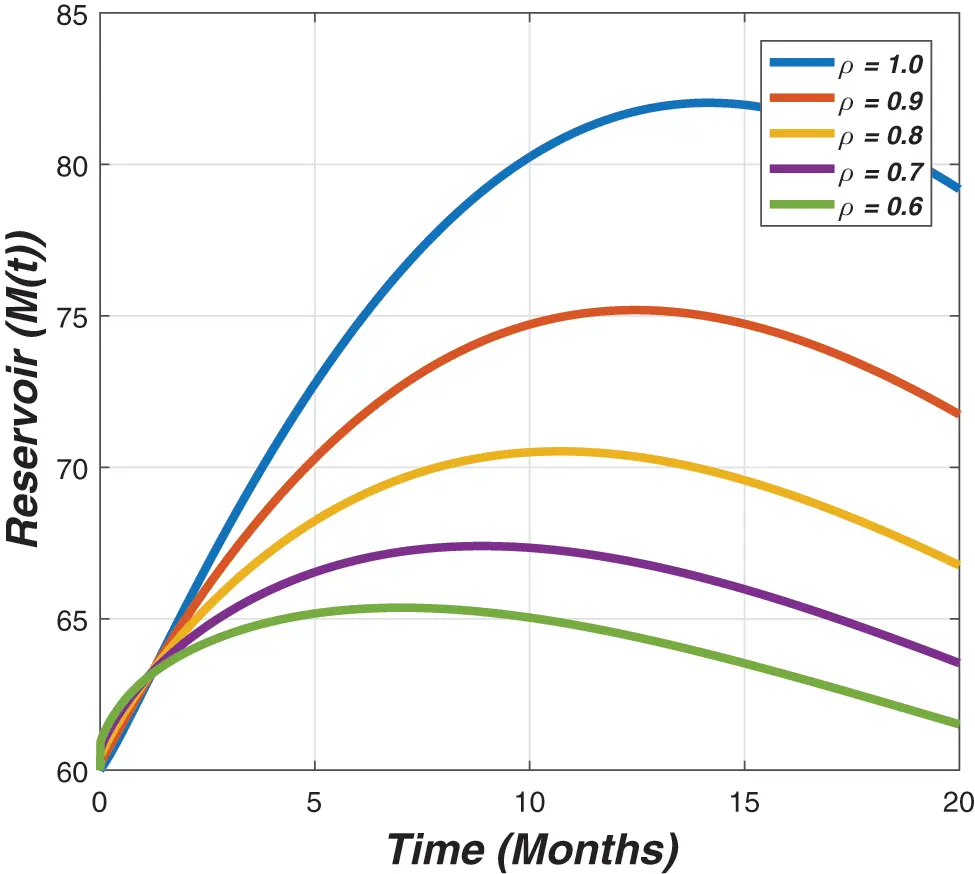Figure 6: The graph describes the dynamics of the ratio of reservoir for different value of the fractional parameter (ρ) and model parameters given in Table 1, while the initial guess are as (100,90,80,70,60)

4  Conclusion

We investigated the dynamics of SARS-CoV-2 with latent and infected individuals using an epidemic model. First, the formulation of the model is proposed and then consequently fractionalized due to the increased development in fractional calculus. Mainly, we used the well-known Caputo-Fabrizio operator for the said purposes, because this operator is based on the non-singular kernel and is more appropriate than the other fractional operator. Moreover, we applied the fixed point theorem to perform the existence analysis with unique properties regarding the developed epidemic problem. The biological and mathematical feasibilities are discussed in detail for the proposed model and prove that the problem is well-possed. Finally, we gave some graphical representations and showed the validations of the obtained results. We also presented the relative impact of the fractional parameter on the various groups of the compartmental populations graphically. We proved that the significant outcome of the reported work is that the fractional-order CF epidemic models are more appropriate and the best choice rather than the classical order.

In the near future, we will use the operators Atangana Baleanu Caputo, Atangana bi order, Atangana Gomez and fractal-fractional operator to study the complex dynamics of novel corona virus disease transmission. We will also apply the optimal control theory to the model reported in this study to present the control mechanism for the novel corona virus disease transmission.

Funding Statement: The author T. Abdeljawad would like to thank Prince Sultan University for the support through the TAS Research Lab and M. A. Alqudah was supported by Princess Nourah bint Abdulrahman University Researchers Supporting Project No. (PNURSP2022R14), Princess Nourah bint Abdulrahman University, Riyadh, Saudi Arabia.

Conflicts of Interest: The authors declare that they have no conflicts of interest to report regarding the present study.

## References

1. Azhar, E. I., El-Kafrawy, S. A., Farraj, S. A., Hassan, A. M., & Al-Saeed, M. S. (2014). Evidence for camel-to-human transmission of mers coronavirus. New England Journal of Medicine, 370(26), 2499-2505. [Google Scholar] [CrossRef]
2. Kim, Y., Lee, S., Chu, C., Choe, S., & Hong, S. (2016). The characteristics of Middle Eastern respiratory syndrome coronavirus transmission dynamics in South Korea. Osong Public Health and Research Perspectives, 7(1), 49-55. [Google Scholar] [CrossRef]
3. Al-Tawfiq, J. A., Hinedi, K., Ghandour, J., Khairalla, H., & Musleh, S. (2014). Middle East respiratory syndrome coronavirus: A case-control study of hospitalized patients. Clinical Infectious Diseases, 59(2), 160-165. [Google Scholar] [CrossRef]
4. Chen, Z., Zhang, W., Lu, Y., Guo, C., Guo, Z. et al. (2020). From SARS-CoV to Wuhan 2019-nCoV outbreak: Similarity of early epidemic and prediction of future trends. CELL-HOST-MICROBE-D-20-00063.
5. Backer, J. A., Klinkenberg, D., & Wallinga, J. (2020). Incubation period of 2019 novel coronavirus (2019-nCoV) infections among travellers from wuhan, China, 20–28 January 2020. Eurosurveillance, 25(5), [Google Scholar] [CrossRef]
6. Chen, S. B., Rashid, S., Noor, M. A., Ashraf, R., & Chu, Y. M. (2020). A new approach on fractional calculus and probability density function. AIMS Mathematics, 5(6), 7041-7054. [Google Scholar] [CrossRef]
7. Abdeljawad, T., Jarad, F., Atangana, A., & Mohammed, P. O. (2020). On a new type of fractional difference operators on h-step isolated time scales: Weighted fractional difference operators. Journal of Fractional Calculus and Nonlinear Systems, 1(1), 46-74. [Google Scholar] [CrossRef]
8. Chen, S. B., Jahanshahi, H., Abba, O. A., Solís-Pérez, J., & Bekiros, S. (2020). The effect of market confidence on a financial system from the perspective of fractional calculus: Numerical investigation and circuit realization. Chaos, Solitons & Fractals, 140, 110223. [Google Scholar] [CrossRef]
9. Al-Mdallal, Q. M., Hajji, M. A., & Abdeljawad, T. (2021). On the iterative methods for solving fractional initial value problems: New perspective. Journal of Fractional Calculus and Nonlinear Systems, 2(1), 76-81. [Google Scholar] [CrossRef]
10. Ziada, E. (2021). Numerical solution for multi-term fractional delay differential equations. Journal of Fractional Calculus and Nonlinear Systems, 2(2), 1-12. [Google Scholar] [CrossRef]
11. Abdeljawad, T., Suwan, I., Jarad, F., & Qarariyah, A. (2021). More properties of fractional proportional differences. Journal of Mathematical Analysis and Modeling, 2(1), 72-90. [Google Scholar] [CrossRef]
12. Rashid, S., Sultana, S., Karaca, Y., Khalid, A., & Chu, Y. M. (2021). Some further extensions considering discrete proportional fractional operators. Fractals, 30, 2240026. [Google Scholar]
13. Atangana, A. (2020). Modelling the spread of COVID-19 with new fractal-fractional operators: Can the lockdown save mankind before vaccination?. Chaos, Solitons & Fractals, 136, 109860. [Google Scholar] [CrossRef]
14. Atangana, A., & Araz, S. I. (2021). Nonlinear equations with global differential and integral operators: Existence, uniqueness with application to epidemiology. Results in Physics, 20, 103593. [Google Scholar] [CrossRef]
15. Haidong, Q., Arfan, M., Salimi, M., Salahshour, S., & Ahmadian, A. (2021). Fractal–fractional dynamical system of typhoid disease including protection from infection. Engineering with Computers, 2021, 1-10. [Google Scholar] [CrossRef]
16. Samko, S. G. (1987). Fractional integrals and derivatives, theory and applications. Minsk: Nauka I Tekhnika.
17. Baleanu, D., Güvenç, Z. B., Machado, J. T. (2010). New trends in nanotechnology and fractional calculus applications. New York: Springer.
18. Baleanu, D., Machado, J. A. T., Luo, A. C. (2011). Fractional dynamics and control. Springer Science & Business Media.
19. Baleanu, D., Diethelm, K., Scalas, E., Trujillo, J. J. (2012). Fractional calculus: Models and numerical methods, vol. 3. World Scientific, Singapore.
20. Ali, N., Zaman, G., Zeb, A., Erturk, V. S., Jung, I. H. et al. (2019). Dynamical analysis of approximate solutions of HIV-1 model with an arbitrary order. Complexity, 7.
21. Zafar, Z. U. A., Ali, N., & Baleanu, D. (2021). Dynamics and numerical investigations of a fractional-order model of toxoplasmosis in the population of human and cats. Chaos, Solitons & Fractals, 151, 111261. [Google Scholar] [CrossRef]
22. Zafar, Z. U. A., Shah, Z., Ali, N., Alzahrani, E. O., & Shutaywi, M. (2021). Mathematical and stability analysis of fractional order model for spread of pests in tea plants. Fractals, 29(1), 2150008. [Google Scholar] [CrossRef]
23. Srivastava, H. M., Saad, K. M., Gómez-Aguilar, J., & Almadiy, A. A. (2020). Some new mathematical models of the fractional-order system of human immune against iav infection. Mathematical Biosciences and Engineering, 17(5), 4942-4969. [Google Scholar] [CrossRef]
24. Tuan, N. H., Mohammadi, H., & Rezapour, S. (2020). A mathematical model for COVID-19 transmission by using the caputo fractional derivative. Chaos, Solitons & Fractals, 140, 110107. [Google Scholar] [CrossRef]
25. Owolabi, K. M., & Atangana, A. (2017). Numerical approximation of nonlinear fractional parabolic differential equations with caputo–fabrizio derivative in riemann–liouville sense. Chaos, Solitons & Fractals, 99, 171-179. [Google Scholar] [CrossRef]
26. Baleanu, D., Jajarmi, A., & Hajipour, M. (2018). On the nonlinear dynamical systems within the generalized fractional derivatives with mittag–leffler kernel. Nonlinear Dynamics, 94(1), 397-414. [Google Scholar] [CrossRef]
27. Baleanu, D., Mohammadi, H., & Rezapour, S. (2020). Analysis of the model of HIV-1 infection of CD4+ T-cell with a new approach of fractional derivative. Advances in Difference Equations, 2020(1), 1-17. [Google Scholar] [CrossRef]
28. Caputo, M., & Fabrizio, M. (2015). A new definition of fractional derivative without singular kernel. Progress in Fractional Differentiation & Applications, 1(2), 73-85. [Google Scholar]
29. Rihan, F., Al-Mdallal, Q., AlSakaji, H., & Hashish, A. (2019). A fractional-order epidemic model with time-delay and nonlinear incidence rate. Chaos, Solitons & Fractals, 126, 97-105. [Google Scholar] [CrossRef]
30. Li, X. P., Al Bayatti, H., Din, A., & Zeb, A. (2021). A vigorous study of fractional order COVID-19 model via abc derivatives. Results in Physics, 29, 104737. [Google Scholar] [CrossRef]
31. Li, X. P., Wang, Y., Khan, M. A., Alshahrani, M. Y., & Muhammad, T. (2021). A dynamical study of SARS-COV-2: A study of third wave. Results in Physics, 29, 104705. [Google Scholar] [CrossRef]
32. Li, X. P., Gul, N., Khan, M. A., Bilal, R., & Ali, A. (2021). A new hepatitis b model in light of asymptomatic carriers and vaccination study through atangana–baleanu derivative. Results in Physics, 29, 104603. [Google Scholar] [CrossRef]
33. Chen, S. B., Soradi-Zeid, S., Jahanshahi, H., Alcaraz, R., & Gómez-Aguilar, J. F. (2020). Optimal control of time-delay fractional equations via a joint application of radial basis functions and collocation method. Entropy, 22(11), 1213. [Google Scholar] [CrossRef]
34. Chen, S. B., Rajaee, F., Yousefpour, A., Alcaraz, R., & Chu, Y. M. (2021). Antiretroviral therapy of hiv infection using a novel optimal type-2 fuzzy control strategy. Alexandria Engineering Journal, 60(1), 1545-1555. [Google Scholar] [CrossRef]
35. Shen, Z. H., Chu, Y. M., Khan, M. A., Muhammad, S., & Al-Hartomy, O. A. (2021). Mathematical modeling and optimal control of the COVID-19 dynamics. Results in Physics, 31, 105028. [Google Scholar] [CrossRef]
36. Jain, S., & El-Khatib, Y. (2021). Stochastic COVID-19 model with fractional global and classical piecewise derivative. Results in Physics, 30, 104788. [Google Scholar] [CrossRef]
37. Shafiq, A., Lone, S., Sindhu, T. N., El Khatib, Y., & Al-Mdallal, Q. M. (2021). A new modified kies fréchet distribution: Applications of mortality rate of COVID-19. Results in Physics, 28, 104638. [Google Scholar] [CrossRef]
38. Rihan, F., & Alsakaji, H. (2021). Dynamics of a stochastic delay differential model for COVID-19 infection with asymptomatic infected and interacting people: Case study in the uae. Results in Physics, 28, 104658. [Google Scholar] [CrossRef]
39. Wu, J. T., Leung, K., & Leung, G. M. (2020). Nowcasting and forecasting the potential domestic and international spread of the 2019-nCoV outbreak originating in Wuhan, China: A modelling study. The Lancet, 395(10225), 689-697. [Google Scholar] [CrossRef]
40. Imai, N., Cori, A., Dorigatti, I., Baguelin, M., Donnelly, C. A. et al. (2020). Report 3: Transmissibility of 2019-nCoV. Imperial College London.
41. Zhu, H., Guo, Q., Li, M., Wang, C., Fang, Z. et al. (2020). Host and infectivity prediction of Wuhan 2019 novel coronavirus using deep learning algorithm. Cold Spring Harbor Laboratory.
42. Rothe, C., Schunk, M., Sothmann, P., Bretzel, G., Froeschl, G. et al. (2020). Transmission of 2019-nCoV infection from an asymptomatic contact in Germany. New England Journal of Medicine, 970–971. DOI 10.1056/NEJMc2001468. [CrossRef]
43. Read, J. M., Bridgen, J. R., Cummings, D. A., Ho, A., Jewell, C. P. (2020). Novel coronavirus 2019-nCoV: Early estimation of epidemiological parameters and epidemic predictions. Cold Spring Harbor Laboratory Press.
44. Li, W., Deng, X., Shao, H., & Wang, X. (2021). Deep learning applications for COVID-19 analysis: A state-of-the-art survey. Computer Modeling in Engineering & Sciences, 129(1), 65-98. [Google Scholar] [CrossRef]
45. Yang, X., Chen, L., & Chen, J. (1996). Permanence and positive periodic solution for the single-species nonautonomous delay diffusive models. Computers & Mathematics with Applications, 32(4), 109-116. [Google Scholar] [CrossRef]
46. Ramos, H., Kalogiratou, Z., Monovasilis, T., & Simos, T. (2016). An optimized two-step hybrid block method for solving general second order initial-value problems. Numerical Algorithms, 72(4), 1089-1102. [Google Scholar] [CrossRef]
47. Li, C., Zeng, F. (2019). Numerical methods for fractional calculus. Chapman and Hall/CRC.

Khan, T., Ullah, R., Abdeljawad, T., Alqudah, M. A., Faiz, F. (2023). A Theoretical Investigation of the SARS-CoV-2 Model via Fractional Order Epidemiological Model. CMES-Computer Modeling in Engineering & Sciences, 135(2), 1295–1313.This work is licensed under a Creative Commons Attribution 4.0 International License , which permits unrestricted use, distribution, and reproduction in any medium, provided the original work is properly cited.

View

Like

## Related articles

• T. Chekifi, B. Dennai, R. Khelfaoui
• N. Soltani, S. Rahal